Members Area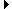Sign Up | Log In
 Free Level 2 QuotesStock AnalysisCorrelation Trading PlatformCorrelationsMembership Benefits

RSI
 Lookup a different indicator.

 Indicator Usage Description Example rsi rsi[x,n] OPERATOR (INDICATOR or NUMBER) , where x is a positive integer >= 2 and n is optional and represents the number of days ago the result is computed (n=0 is the default value and represents the current end of day, n=1 is the previous end of day, etc.) Returns the x-day relative strength index from n days ago. (rsi < 30)

Introduction:

The RSI (relative strength index) is a ratio of the total magnitude of the gains (based on daily closing prices) to the total magnitude of the losses using x-day periods. The RSI is a momentum oscillator (varies between 0-100%). It was introduced by J. Welles Wilder in 1978.

Example:

The following chart of Intel (INTC) highlights days where the 14-day relative strength index is less than 30%.

 Chart of Intel (INTC) highlighting days where the 14-day relative strength index is less than 30%.

INDICATORS IN OUR DATABASE THAT INCLUDE THE RSI INDICATOR...

Click here to view all indicators that include the indicator 'rsi' (sorted by AISCORE)

 (rsi > 30)AISCORE: 2451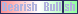Indicator ID: 060424093903(rsi < 30)AISCORE: 5781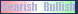Indicator ID: 060423164246(lgbody) and (black) and (rsi < 30)AISCORE: 5343Indicator ID: 060506101239(rsi > 52) and (p1range < 5) and (p1change < -2)AISCORE: 4611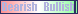Indicator ID: 060811175100(rsi > 14) and (p3change > 1) and (p2change > 2) and (imi > 4)AISCORE: -359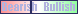Indicator ID: 060811180159 More ->